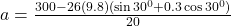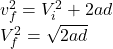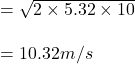## If the motor exerts a constant force of 300 N on the cable, determine the speed of the 26-kg crate when it travels s = 10 m up the plane, st

Question

If the motor exerts a constant force of 300 N on the cable, determine the speed of the 26-kg crate when it travels s = 10 m up the plane, starting from rest. The coefficient of kinetic friction between the crate and the plane is μk = 0.3.

in progress 0
5 months 2021-08-05T01:03:04+00:00 1 Answers 98 views 0

## Answers ( )

10.32m/s

Explanation:

F = ma

T -mg sinθ-f =ma

We can conclude that T = 300 newtons by taking a freebody of the cable. So we need to determine only the frictional force.

f(n) = 0

mgcosθ = N = 0

N = mgcosθ

T – mg sinθ – u mgcosθ = ma

a = T – mg (sinθ + u cosθ) / ma = 5.32m/s^2

Use constant acceleration kinematics:=